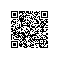# Todd.log - a place to keep my thoughts on programming TF-IDF模型的概率解释

#### tf-idf模型

1) 词w在文档d中的词频tf (Term Frequency)，即词w在文档d中出现次数count(w, d)和文档d中总词数size(d)的比值：

tf(w,d) = count(w, d) / size(d)


2) 词w在整个文档集合中的逆向文档频率idf (Inverse Document Frequency)，即文档总数n与词w所出现文件数docs(w, D)比值的对数:

idf = log(n / docs(w, D))


tf-idf模型根据tf和idf为每一个文档d和由关键词w...w[k]组成的查询串q计算一个权值，用于表示查询串q与文档d的匹配度：

tf-idf(q, d)
= sum { i = 1..k | tf-idf(w[i], d) }
= sum { i = 1..k | tf(w[i], d) * idf(w[i]) }


#### 信息检索问题的概率视角

1) 为什么tf是count(w, d) / size(d)？能不能是log(count(w, d) / size(d))等其他形式？

2) 为什么idf是一个log形式？

3) 为什么tf和idf之间是乘积关系，而不是加法或指数关系？

4) 为什么多个关键词的tf-idf值是加法关系，而不是乘法或者指数关系？

5) 除了tf-idf值，Google还会计算网页的PageRank值，二者相乘得到最后的权值，为什么是乘法，而不是加法或指数？

### 盒子小球模型

P(d[i] | w[j])
= P(d[i], w[j]) / P(w[j])
= P(d[i]) * P(w[j] | d[i]) / P(w[j])


#### 词的信息量和idf

P(d[i] | w[j])
= P(d[i], w[j]) / P(w[j])
= P(d[i]) * P(w[j] | d[i]) / P(w[j])
= P(d[i]) * (tf(w[j], d[i]) * idf(w[j] / sum { k = 1..size(d[i]), tf(w[k], d[i]) * idf(w[k]) }) / p(w[j])
= P(d[i]) * (tf-idf(w[j], d[i]) / sum { k = 1..size(d[i]), tf-idf(w[k], d[i]) }) / p(w[j])
= P(d[i]) * (tf-idf(w[j], d[i]) / tf-idf(d[i])) / p(w[j])


#### 多关键词

P(w[x], w[y]) = P(w[x]) * P(w[y])
P(w[x], w[y] | d[i]) = P(w[x] | d[i]) * P(w[y] | d[i])


P(d[i] | w[x], w[y])
= P(d[i], w[x], w[y]) / P(w[x], w[y])
= P(d[i]) * P(w[x], w[y] | d[i]) / P(w[x], w[y])
= P(d[i]) * P(w[x] | d[i]) * P(w[y] | d[i]) / (P(w[x] * P(w[y]))
= P(d[i]) * (tf-idf(w[x], d[i]) / tf-idf(d[i])) * ((tf-idf(w[y], d[i]) / tf-idf(d[i]))) / (p(w[x]) * P(w[y]))


#### 总结

TF-IDF模型是搜索引擎中广泛使用的信息检索模型，但对于TF-IDF模型一直存在各种疑问。本文为信息检索问题一种基于条件概率的盒子小球模型，其核心思想是把“查询串q和文档d的匹配度问题”转化为“查询串q来自于文档d的条件概率问题”。它从概率的视角为信息检索问题定义了比TF-IDF模型所表达的匹配度更为清晰的目标。从概率模型中，我们看到查询串q来自于文档d的条件概率主要包含以下几个因素：1) 文档的先验概率P(d[i])，这与PageRank对应；2) 词w被作为搜索关键词的先验概率P(w)，这可以通过统计方法获得；3) 关键词w代表文档d主题，或以词w搜索文档d的概率，P(w | d)，除了统计方法，这可以通过tf-idf来计算。使用钉钉扫一扫加入圈子
+ 订阅